Question

# Suppose you want to test the claim the the paired sample data given below come from...

Suppose you want to test the claim the the paired sample data given below come from a population for which the mean difference is μd=0. xy7866678183687180529154928286 Use a 0.01 significance level to find the following: (a) The mean value of the differnces d for the paired sample data d¯= (b) The standard deviation of the differences d for the paired sample data sd= (c) The t test statistic t= (d) The positive critical value t= (e) The negative critical value t= (f) Does the test statistic fall in the critical region? A. No B. Yes (g) Construct a 99% conficence interval for the population mean of all differences x−y. <μd

Computational Table:

 X Y d=x-y d^2 78 66 12 144 67 81 -14 196 83 68 15 225 71 80 -9 81 52 91 -39 1521 54 92 -38 1444 82 86 -4 16 Total -77 3627

a)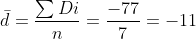b)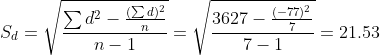c) Test statistic: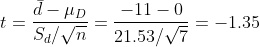d)

Degrees of freedom = n-1 = 7-1 = 6

Critical value: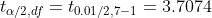...............................From t table

Therefore,

Positive Critical value: 3.7074

Negative Critical value: -3.7074

Conclusion:

Test statistic (t) lies in the rejection region,i.e. -3.7074 < -1.35 < 3.7074, That is Fail to Reject Ho at 1% level of significance.

f) Yes, Test statistic -1.35 is Falls in the critical region

g) Construct 99% Confidence interval: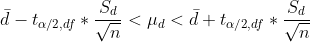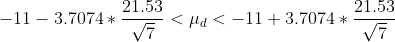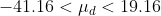We are 99% Confidence that population mean difference is lies in that interval.

#### Earn Coins

Coins can be redeemed for fabulous gifts.

Similar Homework Help Questions
• ### Assume that you want to test the claim that the paired sample data come from a...

Assume that you want to test the claim that the paired sample data come from a population for which the mean difference is μd = 0. Compute the value of the t test statistic. Round intermediate calculations to four decimal places as needed and final answers to three decimal places as needed. A farmer has decided to use a new additive to grow his crops. He divided his farm into 10 plots and kept records of the corn yield (in...

• ### 16) Assume that you want to test the claim that the paired sample data come from...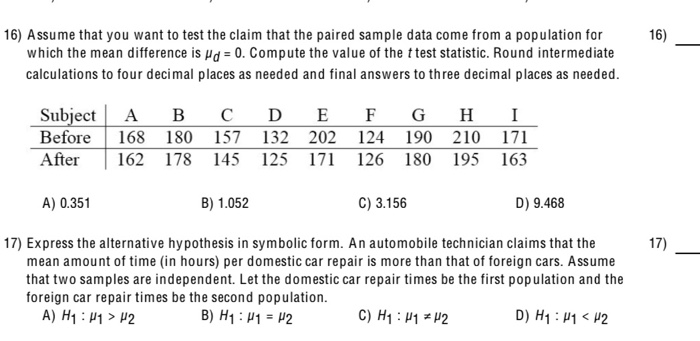16) Assume that you want to test the claim that the paired sample data come from a population for which the mean difference is d = 0. Compute the value of the t test statistic. Round intermediate calculations to four decimal places as needed and final answers to three decimal places as needed. Subject Before After A 168 162 B 180 178 C 157 145 D E 132 202 125 171 F 124 126 G H I 190 210 171...

• ### Test the claim below about the mean of the differences for a population of paired data...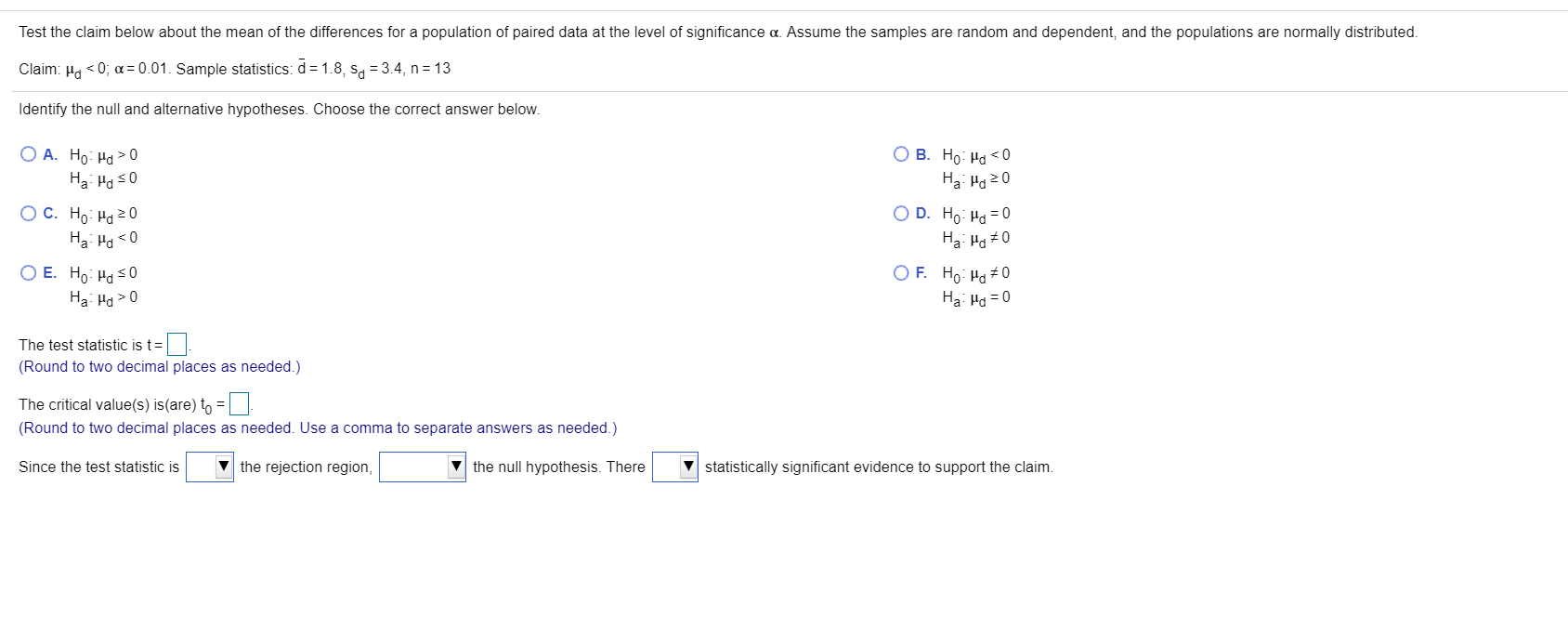Test the claim below about the mean of the differences for a population of paired data at the level of significance a. Assume the samples are random and dependent, and the populations are normally distributed. Claim: Ho<0; a=0.01. Sample statistics: d = 1.8, Sa = 3.4, n = 13 Identify the null and alternative hypotheses. Choose the correct answer below. 0 O B. Ho Hd < 0 Ha Ha 20 OD. Ho Hd = 0 O A. Ho Hd >...

• ### Question 19 4 pts Assume that you want to test the claim that the paired sample...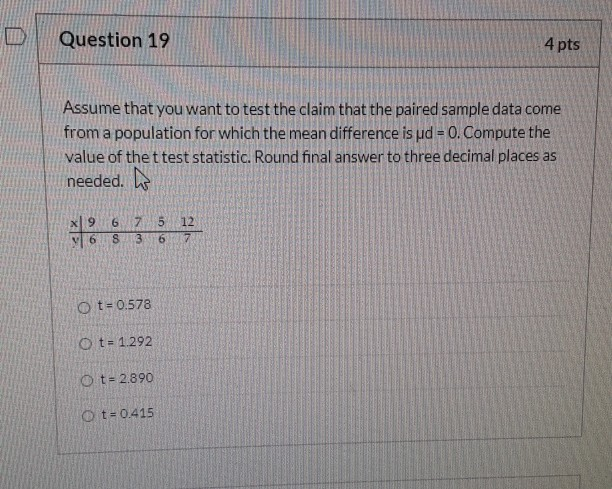Question 19 4 pts Assume that you want to test the claim that the paired sample data come from a population for which the mean difference is ud = 0. Compute the value of the t test statistic. Round final answer to three decimal places as needed. 9 5 7 3 12 7 S 6 O t = 0.578 O t= 1.292 Ot=2.890 O t= 0.415

• ### Test statistic

Assume you want to test the claim that the paired sample data come from a population for which the mean differences is μd = 0. Compute thevalue of the test statistic.Table:x88697y32649

• ### You wish to test the following claim (H1) at a significance level of α=0.002α=0.002. For the...

You wish to test the following claim (H1) at a significance level of α=0.002α=0.002. For the context of this problem, d=x2−x1 where the first data set represents a pre-test and the second data set represents a post-test.       Ho:μd=0       H1:μd≠0 You believe the population of difference scores is normally distributed, but you do not know the standard deviation. You obtain pre-test and post-test samples for n=23 subjects. The average difference (post - pre) is ¯d=−4.1 with a standard deviation of the...

• ### You wish to test the following claim (HAHA) at a significance level of α=0.01α=0.01. For the cont...

You wish to test the following claim (HAHA) at a significance level of α=0.01α=0.01. For the context of this problem, μd=μ2−μ1μd=μ2-μ1 where the first data set represents pre-strike production and the second data set represents post-strike production.       Ho:μd=0Ho:μd=0       HA:μd<0HA:μd<0 You believe the population difference of production is normally distributed, but you do not know the standard deviation. You obtain pre-strike and post-strike production samples for n=6n=6 subjects. The average difference (post - pre) is ¯d=−47.4d¯=-47.4 with a standard deviation of...

• ### You wish to test the following claim (H1) at a significance level of α=0.002α=0.002. For the...

You wish to test the following claim (H1) at a significance level of α=0.002α=0.002. For the context of this problem, d=x2−x1 where the first data set represents a pre-test and the second data set represents a post-test.       Ho:μd=0       H1:μd>0 You believe the population of difference scores is normally distributed, but you do not know the standard deviation. You obtain pre-test and post-test samples for n=22 subjects. The average difference (post - pre) is ¯d=32 with a standard deviation of the...

• ### You wish to test the following claim (HaHa) at a significance level of α=0.05α=0.05. For the...

You wish to test the following claim (HaHa) at a significance level of α=0.05α=0.05. For the context of this problem, μd=μ2−μ1μd=μ2-μ1 where the first data set represents a pre-test and the second data set represents a post-test.       Ho:μd=0Ho:μd=0       Ha:μd>0Ha:μd>0 You believe the population of difference scores is normally distributed, but you do not know the standard deviation. You obtain pre-test and post-test samples for n=8n=8 subjects. The average difference (post - pre) is ¯d=5.2d¯=5.2 with a standard deviation of the...

• ### You wish to test the following claim (H) at a significance level of a = 0.001....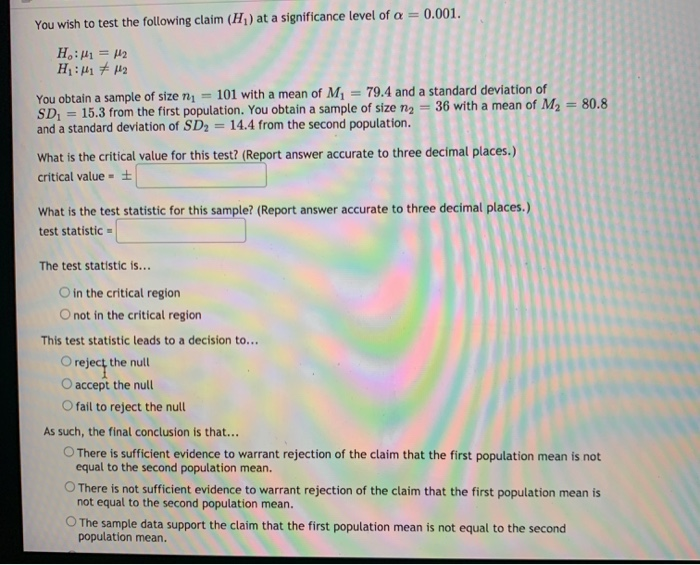You wish to test the following claim (H) at a significance level of a = 0.001. H:41 = M2 H:M 7 H2 You obtain a sample of size m 101 with a mean of M = 79.4 and a standard deviation of SD = 15.3 from the first population. You obtain a sample of size ng = 36 with a mean of M2 = 80.8 and a standard deviation of SD = 14.4 from the second population. What is the...